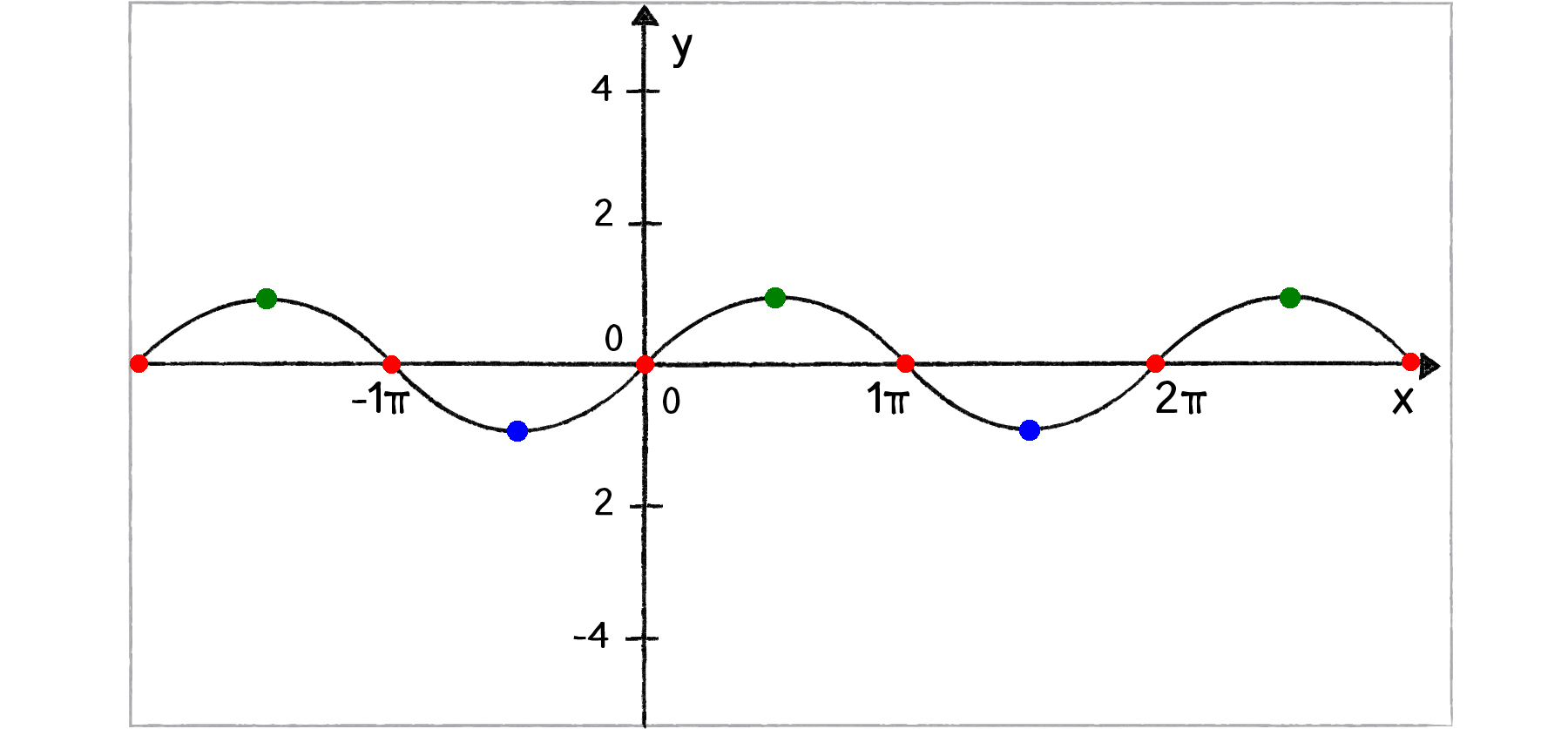# Fun with infinite setsImagine the graph of function the $f(x)=\sin(x).$ The domain of this function is the set of all real numbers $R.$

Consider the three sets

• $A=\left\{ x\in \mathbb R|\sin(x)=-1 \right\}$
• $B=\left\{ x\in \mathbb R|\sin(x)=0 \right\}$
• $C=\left\{ x\in \mathbb R|\sin(x)=1 \right\}.$

Which of them has the largest cardinality?

×